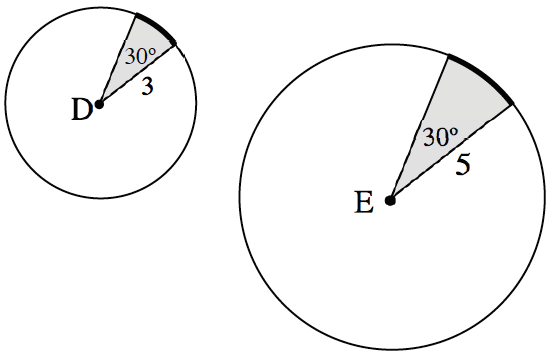### Home > CCG > Chapter 10 > Lesson 10.1.5 > Problem10-55

10-55.Use the sectors in circles $D$ and $E$ to answer the following questions.

1. What is the ratio of the arc length to the radius of circle $D$? Leave your answer in terms of $π$.

The arc length is $\frac{30}{360}\cdot\ 2\pi(3)=\frac{\pi}{2}$.

2. What is the ratio of the arc length to the radius of circle $E$?

You can use the same method as in part (a).

As in part (a), the ratio is $\frac{\pi}{6}$.

3. The ratio of the arc length to the radius of a circle is called a radian. Why will all sectors with a central angle of $30º$ have the same radian measure?

Answers may vary. Example: The circles are similar.